### MROUND Function Examples in Excel, VBA, & Google Sheets

This tutorial demonstrates how to use the Excel MROUND Function in Excel to round a number to a specified multiple.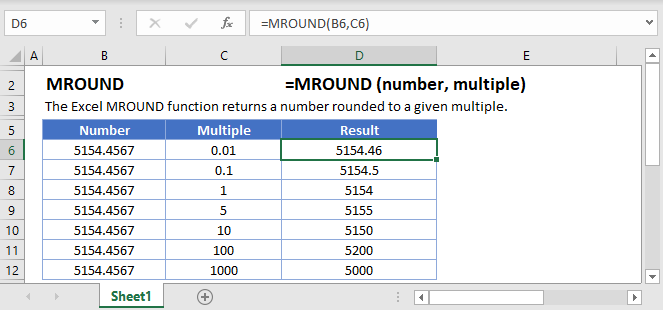## MROUND Function Overview

The MROUND Function Rounds a number to a specified multiple.

To use the MROUND Excel Worksheet Function, select a cell and type: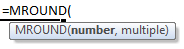(Notice how the formula inputs appear)

### MROUND Function Syntax and Inputs:

`=MROUND(number,multiple)`

number – A number.

multiple – The multiple to which to round the number.

AutoMacro - VBA Code Generator

## How to use the MROUND Function

### Round to Nearest 5, 50, or .5

To round to the nearest 5, set the multiple argument to 5.

`=MROUND(B3,5)`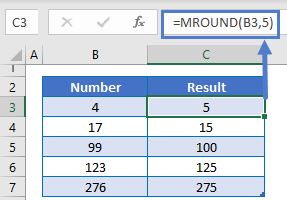Alternatively you can round to the nearest .5 or 50 by changing the multiple argument.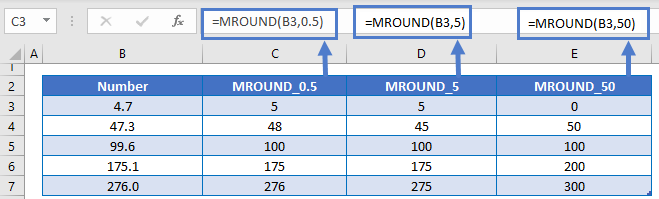### Round to Nearest Quarter

You can also round a price to the nearest quarter by setting multiple = .25.

`=MROUND(A2,0.25)`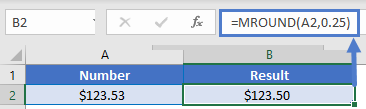### Round Time

The MROUND Function makes it easy to round time. To round time to a certain increment, just enter your desired unit of time using quotations. Here we will round the nearest 15 minutes.

`=MROUND(B3,"0:15")`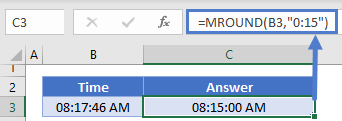## Other ROUND Functions / Formulas

Excel / Google Sheets contains many other round functions. Here are quick examples of each: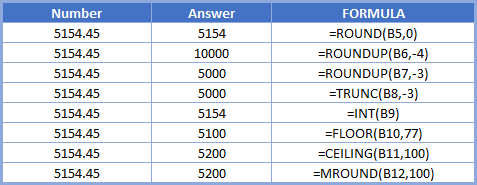Read our tutorials to learn more:

Below, we will point out a few functions in particular.

### FLOOR and CEILING

The FLOOR and CEILING Functions work exactly the same as the MROUND Function, except the FLOOR function always rounds down and the CEILING function always rounds up.

```=MROUND(B3,5)
=FLOOR(B3,5)
=CEILING(B3,5)
```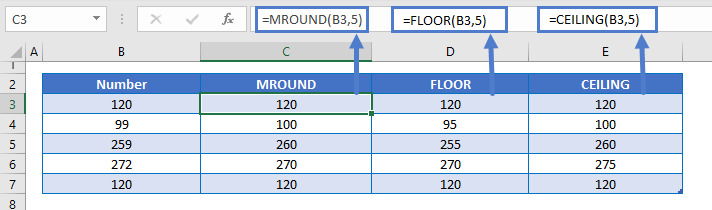### ROUND Function

Instead of using the MROUND Function to round to a multiple, you can use the ROUND Function to round a number to a certain number of digits.

`=ROUND(B3,2)`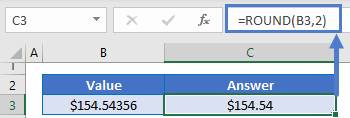## MROUND in Google Sheets

The MROUND Function works exactly the same in Google Sheets as in Excel: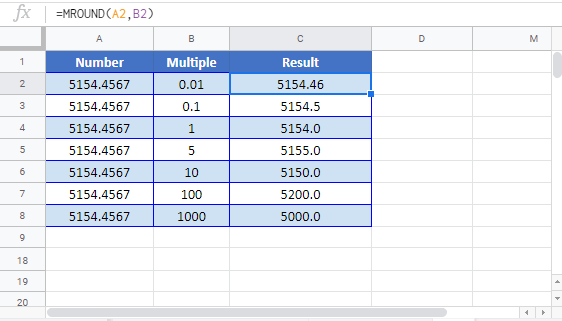## MROUND Examples in VBAYou can also use the MROUND function in VBA. Type:
`Application.Worksheetfunction.Mround(number,multiple)`
For the function arguments (number, etc.), you can either enter them directly into the function, or define variables to use instead.

## Excel Practice Worksheet

Practice Excel functions and formulas with our 100% free practice worksheets!

• Automatically Graded Exercises
• Learn Excel, Inside Excel!